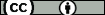# Repositorio Institucional de Documentos

## Boundary layers in a two-point boundary value problem with a caputo fractional derivative

Stynes, M. ; Gracia, J.L. (Universidad de Zaragoza)
Resumen: A two-point boundary value problem is considered on the interval [0, 1], where the leading term in the differential operator is a Caputo fractional derivative of order ¿ with 1 < ¿ < 2. Writing ¿ for the solution of the problem, it is known that typically ¿¿¿(¿) blows up as ¿ ¿ 0. A numerical example demonstrates the possibility of a further phenomenon that imposes difficulties on numerical methods: ¿ may exhibit a boundary layer at ¿ = 1 when ¿ is near 1. The conditions on the data of the problem under which this layer appears are investigated by first solving the constant-coefficient case using Laplace transforms, determining precisely when a layer is present in this special case, then using this information to enlighten our examination of the general variable-coefficient case (in particular, in the construction of a barrier function for ¿). This analysis proves that usually no boundary layer can occur in the solution ¿ at ¿ = 0, and that the quantity ¿ = max¿¿[0,1] ¿(¿), where ¿ is the coefficient of the first-order term in the differential operator, is critical: when¿ < 1,noboundarylayerispresentwhen¿isnear1,butwhen¿ = 1thenaboundarylayerat¿ = 1 is possible. Numerical results illustrate the sharpness of most of our results.
Idioma: Inglés
DOI: 10.1515/cmam-2014-0024
Año: 2015
Publicado en: Computational Methods in Applied Mathematics 15, 1 (2015), 79-95
ISSN: 1609-4840

Factor impacto JCR: 0.673 (2015)
Categ. JCR: MATHEMATICS, APPLIED rank: 163 / 254 = 0.642 (2015) - Q3 - T2
Factor impacto SCIMAGO: 0.901 - Applied Mathematics (Q2) - Numerical Analysis (Q2) - Computational Mathematics (Q2)

Financiación: info:eu-repo/grantAgreement/ES/MEC/MTM2010-16917
Tipo y forma: Artículo (Versión definitiva)Debe reconocer adecuadamente la autoría, proporcionar un enlace a la licencia e indicar si se han realizado cambios. Puede hacerlo de cualquier manera razonable, pero no de una manera que sugiera que tiene el apoyo del licenciador o lo recibe por el uso que hace.

Este artículo se encuentra en las siguientes colecciones:
Artículos

Registro creado el 2016-06-28, última modificación el 2021-01-21